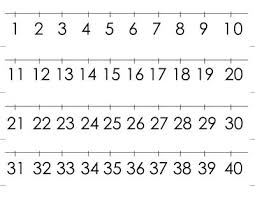# Calculate 65044

The product of the three numbers is 224. The first is 10, and the second is 50 times smaller than the first. Calculate the third number.

c =  112

### Step-by-step explanation:Did you find an error or inaccuracy? Feel free to write us. Thank you!

Tips for related online calculators
Need help calculating sum, simplifying, or multiplying fractions? Try our fraction calculator.